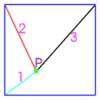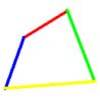# Resources tagged with: Sine rule & cosine rule

Filter by: Content type:
Age range:
Challenge level:

### There are 16 results

Broad Topics > Pythagoras and Trigonometry > Sine rule & cosine rule### Pythagoras for a Tetrahedron

##### Age 16 to 18Challenge Level

In a right-angled tetrahedron prove that the sum of the squares of the areas of the 3 faces in mutually perpendicular planes equals the square of the area of the sloping face. A generalisation. . . .### Square World

##### Age 16 to 18Challenge Level

P is a point inside a square ABCD such that PA= 1, PB = 2 and PC = 3. How big is angle APB ?### Xtra

##### Age 14 to 18Challenge Level

Find the sides of an equilateral triangle ABC where a trapezium BCPQ is drawn with BP=CQ=2 , PQ=1 and AP+AQ=sqrt7 . Note: there are 2 possible interpretations.### 30-60-90 Polypuzzle

##### Age 16 to 18Challenge Level

Re-arrange the pieces of the puzzle to form a rectangle and then to form an equilateral triangle. Calculate the angles and lengths.### Just Touching

##### Age 16 to 18Challenge Level

Three semi-circles have a common diameter, each touches the other two and two lie inside the biggest one. What is the radius of the circle that touches all three semi-circles?### Quadarc

##### Age 14 to 16Challenge Level

Given a square ABCD of sides 10 cm, and using the corners as centres, construct four quadrants with radius 10 cm each inside the square. The four arcs intersect at P, Q, R and S. Find the. . . .### Hexy-metry

##### Age 14 to 16Challenge Level

A hexagon, with sides alternately a and b units in length, is inscribed in a circle. How big is the radius of the circle?### Get Cross

##### Age 14 to 16Challenge Level

A white cross is placed symmetrically in a red disc with the central square of side length sqrt 2 and the arms of the cross of length 1 unit. What is the area of the disc still showing?### Darts and Kites

##### Age 14 to 16Challenge Level

Explore the geometry of these dart and kite shapes!### The Dodecahedron Explained

##### Age 16 to 18

What is the shortest distance through the middle of a dodecahedron between the centres of two opposite faces?### Raising the Roof

##### Age 14 to 16Challenge Level

How far should the roof overhang to shade windows from the mid-day sun?### Biggest Bendy

##### Age 16 to 18Challenge Level

Four rods are hinged at their ends to form a quadrilateral. How can you maximise its area?### Cubestick

##### Age 16 to 18Challenge Level

Stick some cubes together to make a cuboid. Find two of the angles by as many different methods as you can devise.### Cyclic Triangles

##### Age 16 to 18Challenge Level

Make and prove a conjecture about the cyclic quadrilateral inscribed in a circle of radius r that has the maximum perimeter and the maximum area.### Calculating with Cosines

##### Age 14 to 18Challenge Level

If I tell you two sides of a right-angled triangle, you can easily work out the third. But what if the angle between the two sides is not a right angle?### Bendy Quad

##### Age 14 to 16Challenge Level

Four rods are hinged at their ends to form a convex quadrilateral. Investigate the different shapes that the quadrilateral can take. Be patient this problem may be slow to load.